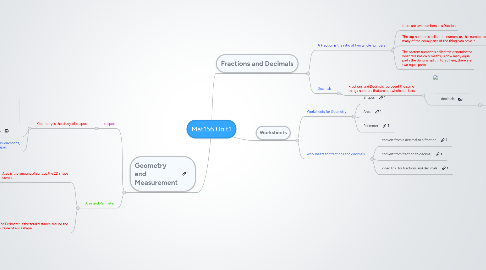# Mat156 Unit1

Get Started. It's Free
or sign up with your email addressMat156 Unit1## 1. Geometry and Measurement

### 1.1. shapes

1.1.1. Geometry is the study of shapes

1.1.1.1. shapes

1.1.1.2. circles, squares, triangles, ovals, diamonds, rectangles are the primary shapes

### 1.2. Area and Perimeter

1.2.1. Area is the amount of surface the 2D shape covers

1.2.1.1. area

1.2.2. The Perimeter is the total distance around the outside of a 2D shape

1.2.2.1. perimeter

## 2. Fractions and Decimals

### 2.1. A Fraction is the ratio of two whole numbers

2.1.1. there are two numbers in a fraction

2.1.2. The top number is called the numerator. this number tells you how many of the equal parts of the thing' you have. t

2.1.3. The bottom number is called the denominator what this basically means, is how many equal parts the thing' is split in to. so here, there are two equal parts.

### 2.2. Decimals

2.2.1. Fractions and Decimals represent the same things numbers that are not whole numbers.

2.2.1.1. decimals

2.2.1.1.1. steps to convert decimals to fractions 1) write down the decimal divided1, like this decimal decimal/1 2)multiply both top and bottom by 10 for every number after the decimal points 3)simplify(or reduce) the fraction

## 3. Worksheets

3.1.1. Shapes

3.1.2. Area

3.1.3. Perimeter

### 3.2. worksheets for fractions and decimals

3.2.1. convert from a decimal to a fraction

3.2.2. convert from fraction to decimal

3.2.3. video link for fractions and decimals